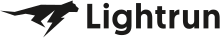• Dev Observability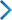• Product• Pricing
• Docs
• Resources• Blog
• Company• Debug Wordle# Stuck on an issue?

Lightrun Answers was designed to reduce the constant googling that comes with debugging 3rd party libraries. It collects links to all the places you might be looking at while hunting down a tough bug.

And, if you’re still stuck at the end, we’re happy to hop on a call to see how we can help out.

## Help with increasing chromaticity diagrams plotting speed.

See original GitHub issue

### Issue Description

I’m back again!

I was frustrated by the slow speed of the chromaticity diagram plotters, so I have begun to re-implement them. I learned that this requires re-writting most of the color space transforms into a numpy ufunc-like format. Versions of the necessary functions that have this format can be found here. Note these versions require that the input be a numpy array rather than an iterable, but the line `var = np.asarray(var)` can be added to the top of each function to remove this requriement. Some additional logic could be added so that e.g. if the input was a list, the output is a list, but this is a detail.

The current plotters, as best I understand, generate a 4000x4000 point scatter plot and color the dots with sRGB tones – matplotlib wasn’t made for plotting 16 million data points quickly!

A more efficient scheme is to make a meshgrid in the desired color space (in my case, u’ v’, though this method is general), “block out” values outside of the horseshoe, and shade with sRGB tones. Additional things like plankian locusts can be added, but the performance issue is with the massive number of scatter points.

Progress towards this can be found here. Unfortunately, a warning is thrown in the XYZ->sRGB conversion; I presume this is because there are imaginary colors present. An image of the result, in sRGB, is below.I would appreciate any help with debugging this and then pulling the changes into `colour`.

timeit results from my laptop with an i7-7700HQ (4c/8t @ 3.6Ghz)

``````%timeit CIE_1976_UCS_chromaticity_diagram_plot(show_diagram_colours=True, standalone=False)
>>>502 ms ± 22.4 ms per loop (mean ± std. dev. of 7 runs, 1 loop each)
``````
``````%%timeit
#from prysm.colorimetry import XYZ_to_xyY, xyY_to_xy, XYZ_to_xy, XYZ_to_uv, Luv_uv_to_xy, xy_to_XYZ
#from prysm.mathops import nan
samples = 256
xlim = (0,1)
ylim = (0,1)

wvl = np.arange(400, 700, 10)
wvl_XYZ = colour.wavelength_to_XYZ(wvl)
wvl_uv = XYZ_to_uv(wvl_XYZ)
wvl_pts = wvl_uv*samples

u = np.linspace(xlim, xlim, samples)
v = np.linspace(ylim, ylim, samples)
uu, vv = np.meshgrid(u, v)

shape = uu.shape

# stack u and v for vectorized computations
uuvv = np.stack((uu,vv), axis=len(shape))

# map -> xy -> XYZ -> sRGB
xy = Luv_uv_to_xy(uuvv)
xyz = xy_to_XYZ(xy)
dat = colour.XYZ_to_sRGB(xyz)
dat_2 = np.swapaxes(dat / dat[np.isfinite(dat)].max(), 0, 1)
plt.imshow(dat_2, origin='lower', extent=[*xlim, *ylim])
plt.gca().set(xlim=(0,0.6),ylim=(0,0.6))
plt.grid('off')
>>> 31.5 ms ± 1.54 ms per loop (mean ± std. dev. of 7 runs, 10 loops each)
``````

Since this includes expensive warning prints, I assume that this will run in closer to 25ms (a 20x improvement!) when it works properly. A 128x128 grid would also be ~4x faster, bringing the time to less than 10ms. This would allow time to budget e.g. expensive lanczos interpolation of the chromatic surface, achieving similar or superior visual quality in greatly reduced time.

What I don’t know, is why I get imaginary colors. Any help in that regard would be appreciated.

### Issue Analytics

• State: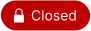• Created 5 years ago

####Top GitHub Comments

4reactions
KelSolaarcommented, Jan 5, 2018

Hi Brandon,

I’m very sorry to see that you are acting like a very young kid.

For reference Colour has been computing Chromaticity Diagram colours using meshgrid for over 2.5 years: https://github.com/colour-science/colour/blob/e9b0ccbbe13db280c8296557ddef572b94415e09/colour/plotting/diagrams.py or here in Colour - Analysis: https://github.com/colour-science/colour-analysis/blob/master/colour_analysis/visuals/diagrams.py#L40

If you take a careful look at our 2.5 years old code you will notice that the `CIE_1931_chromaticity_diagram_colours_plot` definition your original post was complaining about the speed is not part of the public API, people are using `CIE_1931_chromaticity_diagram_plot` which loads a very high resolution image into an array and pass it directly to `pylab.imshow` to avoid jaggies in the background:

``````    image = matplotlib.image.imread(
os.path.join(PLOTTING_RESOURCES_DIRECTORY,
'CIE_1931_Chromaticity_Diagram_{0}.png'.format(
cmfs.name.replace(' ', '_'))))
pylab.imshow(image, interpolation='nearest', extent=(0, 1, 0, 1))
``````

Your great idea (remember one cannot copyright ideas) is to pass an RGB array directly to `pylab.imshow`. Now with that in mind can you please show us the lines of code we copied from you, I bet you will have a very hard time because even the example I pasted in this thread here: https://github.com/colour-science/colour/issues/362#issuecomment-347101656 is not in use in Colour.

By the way, should I ask you to attribute the fixes I did to your implementation under the New BSD License?

It’s now 2:30am my time so I’ll be a bit brief, but I looked over your code and made the same change (normalize before tacking on the alpha channel) and the plot is now correct! 😄

Now something we do, and pretty much no one else does is giving people attribution if they participate in issues discussions which is why you are listed in the following locations:

``````## colour.plotting
- `colour.plotting.CIE_1931_chromaticity_diagram_plot`: (@brandondube, @kelsolaar)
- Name: `chromaticity_diagram_plot_CIE1931`
- Signature: `chromaticity_diagram_plot_CIE1931(cmfs='CIE 1931 2 Degree Standard Observer', show_diagram_colours=True, use_cached_diagram_colours=True, **kwargs)`
- `colour.plotting.CIE_1960_UCS_chromaticity_diagram_plot`: (@brandondube, @kelsolaar)
- Name: `chromaticity_diagram_plot_CIE1960UCS`
- Signature: `chromaticity_diagram_plot_CIE1960UCS(cmfs='CIE 1931 2 Degree Standard Observer', show_diagram_colours=True, use_cached_diagram_colours=True, **kwargs)`
- `colour.plotting.CIE_1976_UCS_chromaticity_diagram_plot`: (@brandondube, @kelsolaar)
- Name: `chromaticity_diagram_plot_CIE1976UCS`
- Signature: `chromaticity_diagram_plot_CIE1976UCS(cmfs='CIE 1931 2 Degree Standard Observer', show_diagram_colours=True, use_cached_diagram_colours=True, **kwargs)`
``````

I’m happy to give a reference to yourself and this thread in the module itself but since we did not copied any of your code, we don’t have to do anything regarding licensing.

1reaction
brandondubecommented, Jan 5, 2018

I ask that code derived from mine be properly attributed under the MIT license, or removed from colour. Intellectually, expression of computing the background image colours directly on the grid is mine; using delaunay instead of a custom function for the same purpose does not distinguish the two.

####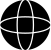Top Results From Across the Web

ChromaticityPlot - Wolfram Language Documentation
ChromaticityPlot is also known as chromatic diagram. · Typically used to visualize one or several color spaces compared to the visual spectrum. ·...
colour.plotting.diagrams.plot_sds_in_chromaticity_diagram
Plots given spectral distribution chromaticity coordinates into the Chromaticity Diagram using given method. Parameters. sds (array_like or ...
Color Part 1: CIE Chromaticity and Perception - ClarkVision.com
The CIE chromaticity diagram (Figure 1) is computed by integrating the X, Y, and Z functions times the object's spectral response function over ......
Colors: CIE XYZ model - Chromaticity graph - Stack Overflow
You may want to increase the luminance by multiplying all the numbers by a constant or something first. Set the pixels at the...
FAQ-444 What is Speed Mode and how does that ... - OriginLab
The Speed Mode property is found in the Plot Details dialog box at the ... layer) to increase redraw speed and decrease the...

####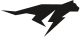Troubleshoot Live Code

Lightrun enables developers to add logs, metrics and snapshots to live code - no restarts or redeploys required.
Start Free

####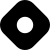Top Related Hashnode Post